.背景知识：

word2vec是一个用来产生词向量的相关模型，使用固定长度(长度较短)的词向量来代替one-hot向量，从而降低深度学习网络中的运算复杂度。其基本思想是使用skip-gram网络/cbow网络来对语料进行训练，留下其中间过程所生成的权重矩阵作为词向量表。这个词向量表是一个[词汇量大小*词向量维度]的矩阵，在使用时，可以使用one-hot向量和词向量表做矩阵乘法，提取出对应词汇的词向量以供后续使用。

.建模思路：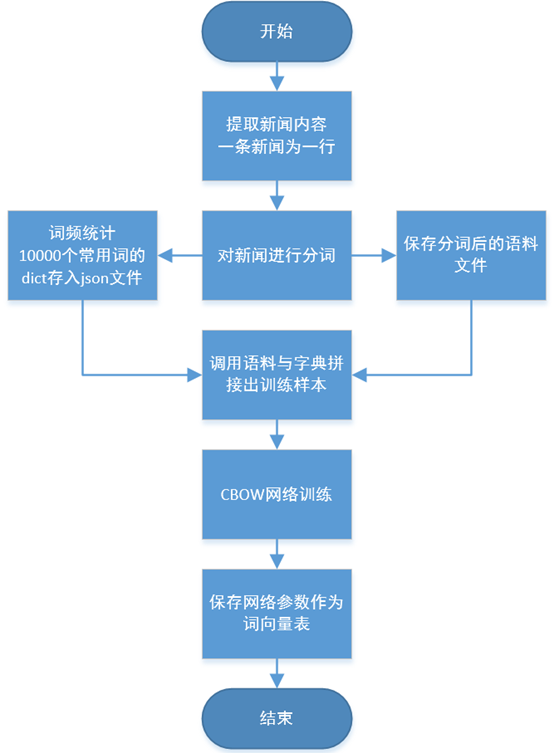.语料预处理：

3.1 乱码问题

 1 pure_file = open(path[:-4]+"_pure.txt",'w',encoding='utf-8')
2 with codecs.open(path,'rb') as f_corp:
3    # TODO 去除杂质编码
4     count = 0    # 记录总共处理了多少行新闻文件
5     count1 = 0   # 记录通过非GBK编码处理了多少行新闻文件
7     for word in lines:
8         try:
9             count += 1
10             # 此处不设置 errors='ignore' 参数，尝试使用GBK解码，遇到解析问题时跳入except处理流程。
11             word_out = word.decode('gbk')
12             pure_file.write(word_out)
13         except:
14             count1 += 1
15             code_name = chardet.detect(word)
16             if code_name['confidence']>0.90:
17                 word_out = word.decode(code_name['encoding'],errors='ignore')
18                 pure_file.write(word_out)
19             else:
20                 continue
21 pure_file.close()

1 content_file = open(path[:-4]+"_content.txt",'w',encoding='utf-8')
2 with open(path,'r',encoding='utf-8') as f_corp:
4     for item in content_lines:
5         if item[:9]=='<content>' and len(item)>20:     # 需判断长度，防止有<content></content> 的情形出现
6             content_file.write(item[9:-11]+'\n')
7 content_file.close()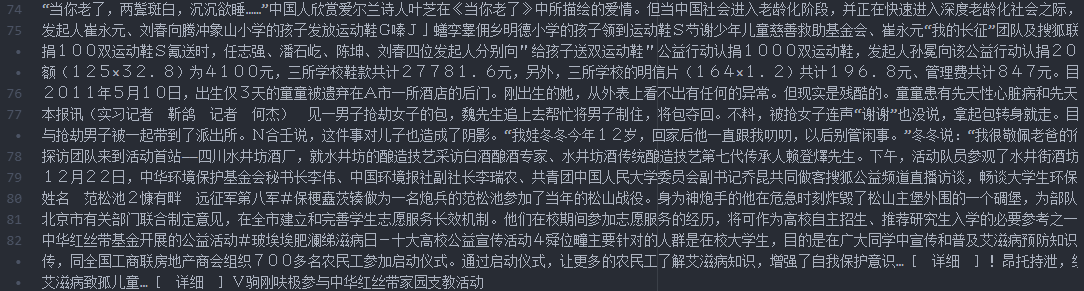3.2 分词与词频统计

1 for ss in c_lines:
2     sentences = ''.join(ch for ch in ss if category(ch)!='P')

1 words = jieba.cut(sentences,cut_all=False)
2 words = ' '.join(words)  # 使用 空格 将每一个词汇隔开 此时是str类型变量。
3 array_c.append(words[:-1])   # 去除句末的 '\n'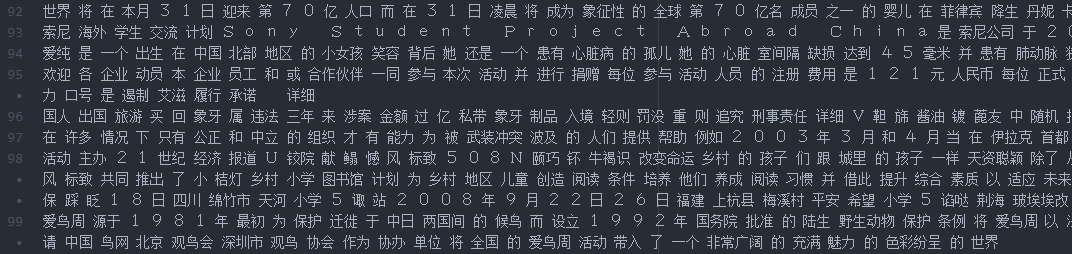1 dict_list_index_last = np.array(array_dn)
2 word_frequent_list = np.argsort(-dict_list_index_last)    # 降序排序，并获取其排序的索引顺序
3 d_out = dict()            # TODO 最终常用词词典列表
4 count = 0
5 for index in word_frequent_list[:10000]:    # 提取10000个常用词汇
6     d_out[array_di[index]] = count
7     count += 1
8 with open(pured_file[:-4]+'_dict.json','w',encoding='utf-8') as f_dict:
9     json.dump(d_out, f_dict)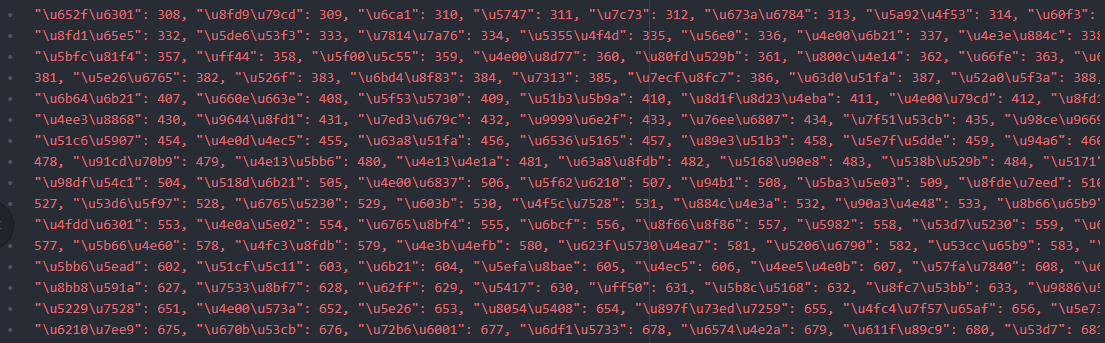.网络搭建：

4.1 模型结构设计

CBOW构造一个训练样本时，样本的输入为当前词汇的前n个词和后n个词，其中n表示窗口长度。例如对于句子[寒冷的 冬天 我 爱 在 学校 里 跑步]，当窗口长度为2的时候，这个句子可以分解为4个训练样本，即[[寒冷的,冬天,爱,在],[我]]，[[冬天,我,在,学校],[爱]]，[[我,爱,学校,里],[在]]，[[爱,在,里,跑步],[学校]]。其中每一个样本的前半部分为输入，后半部分为其对应的输出。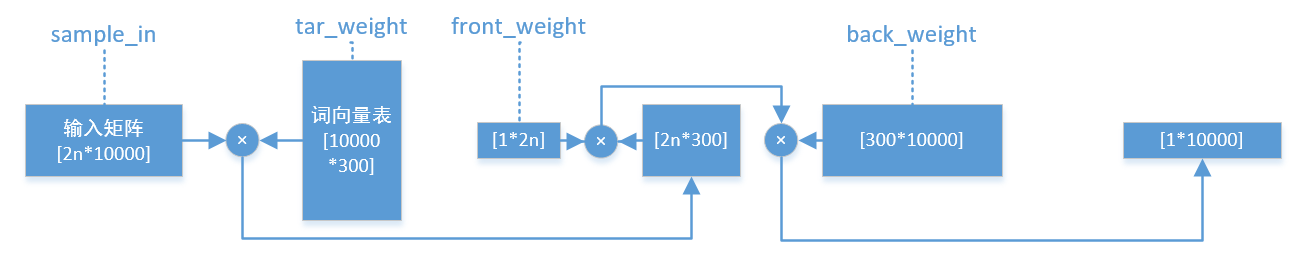1 import numpy as np
2 import tensorflow as tf
3
4 class CBOW_Cell(object):
5     def __init__(self, window_length=5, word_dim=300):
6         with tf.variable_scope("matrix_scope") as matrix_scope:
7             self.tar_weight = tf.get_variable(name='tar_weight',shape=[10000,word_dim],\
8                 initializer=tf.truncated_normal_initializer(stddev=0.1),dtype=tf.float32)
9             self.front_weight = tf.get_variable(name='front_weight',shape=[1,2*window_length],\
10                 initializer=tf.truncated_normal_initializer(stddev=0.1),dtype=tf.float32)
11             self.back_weight = tf.get_variable(name='back_weight',shape=[word_dim,10000],\
12                 initializer=tf.truncated_normal_initializer(stddev=0.1),dtype=tf.float32)
13             matrix_scope.reuse_variables()
14         # 上方为tar_weight,front_weight,back_weight 三个权重矩阵的维度设置及初始化。
15         # 下方为偏移量权重的设置 及 变量保存。
16         self.bias = tf.Variable(tf.zeros([1,10000])) # 偏移量，用于加到softmax前的输出上
17         self.word_dim = word_dim   # 词向量维度
18         self.window_length = window_length
19         # 下方为占位符，规定好输入、输出的shape
20         self.sample_in = tf.placeholder(tf.float32, [2*window_length, 10000],name='sample_in')
21         self.sample_out = tf.placeholder(tf.float32, [1, 10000],name='sample_out')

 1     def forward_prop(self,s_input):
2         step_one = tf.matmul(s_input,self.tar_weight)
3         out_vector = tf.matmul(tf.matmul(self.front_weight,step_one),self.back_weight)+self.bias
4         return out_vector
5
6     def loss_func(self,lr=0.001):
7         out_vector = self.forward_prop(self.sample_in)
8         y_pre = tf.nn.softmax(out_vector,name='y_pre')
9         cross_entropy = tf.nn.softmax_cross_entropy_with_logits_v2(labels=self.sample_out,logits=y_pre)
11         return y_pre,cross_entropy,train_op

4.2 制作训练样本

 1 def make_samples(crop_lines_all,index_to_word,word_to_index,window_len,i):   #参数中的i指第几轮语料
2     # 一次处理5行语料防止内存溢出
3     crop_lines = crop_lines_all[i*5:(i+1)*5]
4     sample_in_list = []     # 输入样本list
5     sample_out_list = []    # 输出样本list
6     for line in crop_lines:
7         line_list = line.split(' ')
8         line_list = [word for word in line_list if word in index_to_word]
9         if len(line_list)<window_len*2+1:     # 如果语句词汇过少，则抛弃这条语句
10             continue
11         else:
12             # 词语大于双倍窗口的情况下，可以开始拼接样本
13             for i2 in range(len(line_list)):
14                 # 句子开头几个词语，前侧的词语数量不够window_len，则后侧多取一些词语攒齐2*window_len的长度
15                 if i2<window_len+1:
16                     temp_line_list = line_list[:i2]+line_list[i2+1:2*window_len+1]
17                     sample_in_list.append(input_matrix_calc(word_to_index,temp_line_list))
18                     temp_out_sample = np.zeros(10000)
19                     temp_out_sample[word_to_index[line_list[i2]]] = 1.0
20                     sample_out_list.append(temp_out_sample)
21                 # 句子末尾几个词语，后侧的词语数量不够window_len，则前侧多取一些词语攒齐2*window_len的长度
22                 elif i2>=len(line_list)-window_len:
23                     temp_line_list = line_list[len(line_list)-2*window_len-1:i2]+line_list[i2+1:]
24                     sample_in_list.append(input_matrix_calc(word_to_index,temp_line_list))
25                     temp_out_sample = np.zeros(10000)
26                     temp_out_sample[word_to_index[line_list[i2]]] = 1.0
27                     sample_out_list.append(temp_out_sample)
28                 # 处于中间阶段，前窗口和后窗口都不越界
29                 else:
30                     temp_line_list = line_list[i2-window_len:i2]+line_list[i2+1:i2+1+window_len]
31                     sample_in_list.append(input_matrix_calc(word_to_index,temp_line_list))
32                     temp_out_sample = np.zeros(10000)
33                     temp_out_sample[word_to_index[line_list[i2]]] = 1.0
34                     sample_out_list.append(temp_out_sample)
35     return np.array(sample_in_list),np.array(sample_out_list)

.训练存储：

 1 def train_model(self, savepath,crop_lines_all,index_to_word,word_to_index,epochs=1000,lr=0.001):
2         y_pre,cross_entropy,train_op = self.loss_func(lr)  # TODO TODO TODO  这句话千万不能放到循环里面，会重复绘制计算图！！！运行很慢！！
3         with tf.Session() as sess:
4             sess.run(tf.global_variables_initializer())
5             for data_num in range(int(len(crop_lines_all)/5)):
6                 pass # 生成 in_list out_list
7                 in_list,out_list = make_samples(crop_lines_all,index_to_word,\
8                     word_to_index,self.window_length,data_num)   #一次20个行的处理语料样本
9                 out_list = out_list.reshape(len(in_list),1,10000)
10                 if (data_num)%50==0:
11                     print('样本已处理',data_num*5,'/',len(crop_lines_all),'行。 ',datetime.datetime.now().strftime('%H:%M:%S.%f'))
12                 for i in range(epochs):
13                     for j in range(len(in_list)):
14                         sess.run(train_op, feed_dict={self.sample_in:in_list[j], \
15                             self.sample_out:out_list[j]})
16         #下面为存储模型的代码
17             tar_weight=self.tar_weight.eval()   # 这个就是词向量表[10000*词向量维度]，是word2vec的最终目标
18             front_weight=self.front_weight.eval()
19             back_weight=self.back_weight.eval()
20             bias=self.bias.eval()
21             word_dim=self.word_dim
22             window_length=self.window_length
23             np.savez(savepath,tar_weight=tar_weight,front_weight=front_weight,\
24                 back_weight=back_weight,bias=bias,word_dim=word_dim,window_length=window_length)
25             print('model saved in:',savepath)

.实践与结果验证：

6.1 词向量表调用：

param_dict = np.load(filepath)
tar_weight = param_dict['tar_weight']


dist = np.linalg.norm(w2v[word_to_index['车辆']] - w2v[word_to_index['车子']])
print('\"车辆\" 与 \"车子\" 之间的欧式距离为：',dist,'!!')
dist = np.linalg.norm(w2v[word_to_index['机械']] - w2v[word_to_index['工业化']])
print('\"机械\" 与 \"工业化\" 之间的欧式距离为：',dist,'!!')
dist = np.linalg.norm(w2v[word_to_index['车辆']] - w2v[word_to_index['茶叶']])
print('\"车辆\" 与 \"茶叶\" 之间的欧式距离为：',dist,'!!')
dist = np.linalg.norm(w2v[word_to_index['粮食']] - w2v[word_to_index['手表']])
print('\"粮食\" 与 \"手表\" 之间的欧式距离为：',dist,'!!')


6.2 效果呈现：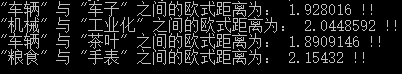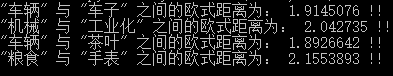.后记

https://mp.weixin.qq.com/s/u2IumPRlzr4uHStrWXM87A

http://www.dataguru.cn/article-13488-1.html